# Five PMP® Exam Formulas Explained

Sep. 21, 2008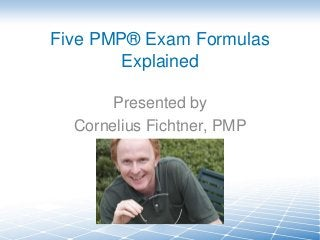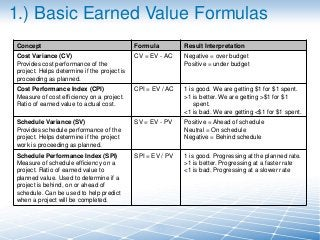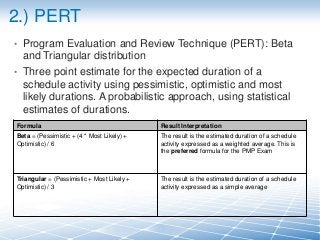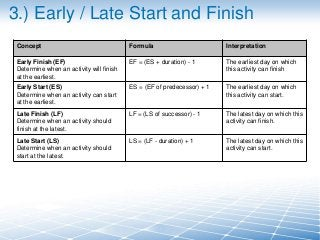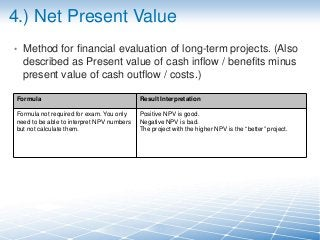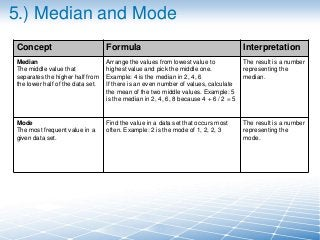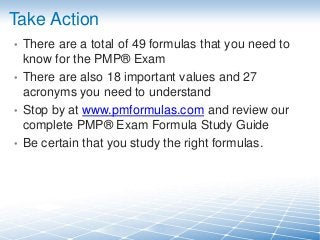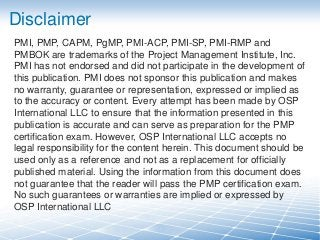1 of 8

### Five PMP® Exam Formulas Explained

• 1. Five PMP® Exam Formulas Explained Presented by Cornelius Fichtner, PMP
• 2. 1.) Basic Earned Value Formulas Concept Formula Result Interpretation Cost Variance (CV) Provides cost performance of the project. Helps determine if the project is proceeding as planned. CV = EV - AC Negative = over budget Positive = under budget Cost Performance Index (CPI) Measure of cost efficiency on a project. Ratio of earned value to actual cost. CPI = EV / AC 1 is good. We are getting \$1 for \$1 spent. >1 is better. We are getting >\$1 for \$1 spent. <1 is bad. We are getting <\$1 for \$1 spent. Schedule Variance (SV) Provides schedule performance of the project. Helps determine if the project work is proceeding as planned. SV = EV - PV Positive = Ahead of schedule Neutral = On schedule Negative = Behind schedule Schedule Performance Index (SPI) Measure of schedule efficiency on a project. Ratio of earned value to planned value. Used to determine if a project is behind, on or ahead of schedule. Can be used to help predict when a project will be completed. SPI = EV / PV 1 is good. Progressing at the planned rate. >1 is better. Progressing at a faster rate <1 is bad. Progressing at a slower rate
• 3. 2.) PERT • • Program Evaluation and Review Technique (PERT): Beta and Triangular distribution Three point estimate for the expected duration of a schedule activity using pessimistic, optimistic and most likely durations. A probabilistic approach, using statistical estimates of durations. Formula Result Interpretation Beta = (Pessimistic + (4 * Most Likely) + Optimistic) / 6 The result is the estimated duration of a schedule activity expressed as a weighted average. This is the preferred formula for the PMP Exam Triangular = (Pessimistic + Most Likely + Optimistic) / 3 The result is the estimated duration of a schedule activity expressed as a simple average
• 4. 3.) Early / Late Start and Finish Concept Formula Interpretation Early Finish (EF) Determine when an activity will finish at the earliest. EF = (ES + duration) - 1 The earliest day on which this activity can finish Early Start (ES) Determine when an activity can start at the earliest. ES = (EF of predecessor) + 1 The earliest day on which this activity can start. Late Finish (LF) Determine when an activity should finish at the latest. LF = (LS of successor) - 1 The latest day on which this activity can finish. Late Start (LS) Determine when an activity should start at the latest. LS = (LF - duration) + 1 The latest day on which this activity can start.
• 5. 4.) Net Present Value • Method for financial evaluation of long-term projects. (Also described as Present value of cash inflow / benefits minus present value of cash outflow / costs.) Formula Result Interpretation Formula not required for exam. You only need to be able to interpret NPV numbers but not calculate them. Positive NPV is good. Negative NPV is bad. The project with the higher NPV is the “better” project.
• 6. 5.) Median and Mode Concept Formula Interpretation Median The middle value that separates the higher half from the lower half of the data set. Arrange the values from lowest value to highest value and pick the middle one. Example: 4 is the median in 2, 4, 6 If there is an even number of values, calculate the mean of the two middle values. Example: 5 is the median in 2, 4, 6, 8 because 4 + 6 / 2 = 5 The result is a number representing the median. Mode The most frequent value in a given data set. Find the value in a data set that occurs most often. Example: 2 is the mode of 1, 2, 2, 3 The result is a number representing the mode.
• 7. Take Action • There are a total of 49 formulas that you need to know for the PMP® Exam • There are also 18 important values and 27 acronyms you need to understand • Stop by at www.pmformulas.com and review our complete PMP® Exam Formula Study Guide • Be certain that you study the right formulas.
• 8. Disclaimer PMI, PMP, CAPM, PgMP, PMI-ACP, PMI-SP, PMI-RMP and PMBOK are trademarks of the Project Management Institute, Inc. PMI has not endorsed and did not participate in the development of this publication. PMI does not sponsor this publication and makes no warranty, guarantee or representation, expressed or implied as to the accuracy or content. Every attempt has been made by OSP International LLC to ensure that the information presented in this publication is accurate and can serve as preparation for the PMP certification exam. However, OSP International LLC accepts no legal responsibility for the content herein. This document should be used only as a reference and not as a replacement for officially published material. Using the information from this document does not guarantee that the reader will pass the PMP certification exam. No such guarantees or warranties are implied or expressed by OSP International LLC